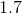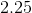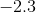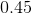# AP Computer Science A : Double

## Example Questions

### Example Question #22 : Primitive Data Types

What line number makes the following Python Code not execute? (1:, 2:, 3:,... represents line numbers)

1: x=[]

2: y=[]

3: z=[]

4: for i in range(10):

5:      x.append(i)

6:      y.append(x[i]+2*x[i])

7:      z.append(x[i]+y[i]**2)

8: print(z)

9: string="The fourth element of z is "+z

10: print(string)

Line 9

Line 7

Line 5

Line 6

Line 8

Line 9

Explanation:

When the code is executed it will produce a type error with the variable string in line 9. The variable string is trying to concatenate a string and an integer from the z array. To make the code executable, we would need to make the integer from the z array a string. We would do this by simply wrapping it in the method str(), which converts it into a string. After making the change, the following code will execute correctly.

1: x=[]

2: y=[]

3: z=[]

4: for i in range(10):

5:      x.append(i)

6:      y.append(x[i]+2*x[i])

7:      z.append(x[i]+y[i]**2)

8: print(z)

9: string="The fourth element of z is"+str(z)

10: print(string)

### Example Question #71 : Computer Science

Consider the following code:

double a = 4.5, d = 4;

int b = 10,c=5;

double e = a / b + c / d - d % c;

What is the value of e at the end of this code's execution?There is a type-related error in the code.Explanation:

The easiest way to work through this code is to comment on its parts. See the comments below in bold.

double a = 4.5, d = 4;

int b = 10,c=5;

/*

a / b: This is a division that will maintain the decimal portion (since it has a double involved in it). 4.5 / 10 will evaluate to 0.45.

c / d: Since this has both an int and a double, it will evaluate to a double. That means that it will maintain its decimal portion as well. It is: 5 / 4 or 1.25.

d % c: Since d is 4, this kind of remainder division works just like having two integers for the modulus. 4 % 5 is just 4. (It is 0 remainder 4.)

Thus, the expression is: 0.45 + 1.25 - 4, which is -2.3.

*/

double e = a / b + c / d - d % c;

### Example Question #23 : Primitive Data Types

What is the size of the float data type in Java?

2 bytes (16 bits)

4 bytes (32 bits)

6 bytes (48 bits)

8 bytes (64 bits)

1 byte (8 bits)

4 bytes (32 bits)

Explanation:

A float is represented by 4 bytes (32 bits). It's comprised of 1 sign bit, 8 exponent bits, and 23 mantissa bits. An example of a floating point number in binary would be 11111111111111111111111111111111. The breakdown would be (sign bit) (exponent bits) (mantissa bits). All in all, that equals 32 bits.

In Java, the byte is the only 8 bit data type. The short and char are 16 bits in size. There are no 48 bit data types, as everything is in a power of 2. The double and long are 64 bits in size.

Because floats have half the number of bits as doubles, it isn't as precise, so shouldn't be used when lots of precision is needed. It's better suited for when there are memory size concerns.

### Example Question #24 : Primitive Data Types

Breanna wants to keep track of how many grapes she eats in a day over the course of a week.

Her brother Nathan, however, wants to keep track of the average amount of grapes he eats in a day over the course of a week.

What type of variable would best be fit for what Breanna wants to keep track of? What about Nathan?

Breanna should use int

Nathan should use double

Breanna should use double

Nathan should use double

Breanna should use double

Nathan should use int

Breanna should use int

Nathan should use int